# Top Python Libraries For Data Science And Machine Learning

• 2144

Data Science and Machine Learning are the most in-demand technologies of the era. This demand has pushed everyone to learn the different libraries and packages to implement Data Science and Machine Learning

This blog post will focus on the Python libraries for Data Science and Machine Learning. These are the libraries you should know to master the two most hyped skills in the market.

Here’s a list of topics that will be covered in this blog:

1. Introduction To Data Science And Machine Learning
2. Why Use Python For Data Science And Machine Learning?
3. Python Libraries for Data Science And Machine Learning
• Python libraries for Statistics
• Python libraries for Visualization
• Python libraries for Machine Learning
• Python libraries for Deep Learning
• Python libraries for Natural Language Processing

# Introduction To Data Science And Machine Learning

When I started my research on Data Science and Machine Learning, there was always this question that bothered me the most! What led to the buzz around Machine Learning and Data Science?

This buzz has a lot to do with the amount of data that we’re generating. Data is the fuel needed to drive Machine Learning models and since we’re in the era of Big Data it is clear why Data Science is considered the most promising job role of the era!I would say that Data Science and Machine Learning are skills, and not just technologies. They are the skills needed to derive useful insights from data and solve problems by building predictive models.

Formally speaking, this is how Data Science and Machine Learning is defined:

Data Science is the process of extracting useful information from data in order to solve real-world problems. Machine Learning is the process of making a machine learn how to solve problems by feeding it lots of data.

These two domains are heavily interconnected. Machine Learning is a part of Data Science that makes use of Machine Learning algorithms and other statistical techniques to understand how data is affecting and growing a business.

Now let’s understand where Python libraries fit into Data Science and Machine Learning.

# Why Use Python For Data Science & Machine Learning?

Python is ranked at number 1 for the most popular programming language used to implement Machine Learning and Data Science. Let’s understand why so many Data Scientists and Machine Learning Engineers prefer Python over any other programming language.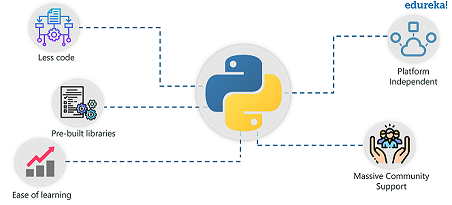• Ease of learning: Python uses a very simple syntax that can be used to implement simple computations like, the addition of two strings to complex processes such as building complex Machine Learning models.
• Less Code: Implementing Data Science and Machine Learning involve tons and tons of algorithms. Thanks to Pythons support for pre-defined packages, we don’t have to code algorithms. And to make things easier, Python provides “check as you code” methodology that reduces the burden of testing the code.
• Prebuilt Libraries: Python has 100s of pre-built libraries to implement various Machine Learning and Deep Learning algorithms. So every time you want to run an algorithm on a data set, all you have to do is install and load the necessary packages with a single command. Examples of pre-built libraries include NumPy, Keras, Tensorflow, Pytorch, and so on.
• Platform Independent: Python can run on multiple platforms including Windows, macOS, Linux, Unix, and so on. While transferring code from one platform to the other you can make use of packages such as PyInstaller that will take care of any dependency issues.
• Massive Community Support: Apart from a huge fan following, Python has multiple communities, groups, and forums where programmers post their errors and help each other.

Now that you know why Python is considered to be one of the best programming languages for Data Science and Machine Learning, let’s understand the different Python libraries for Data Science and Machine Learning.

# Python Libraries For Data Science And Machine Learning

The single most important reason for the popularity of Python in the field of AI and Machine Learning is the fact that Python provides 1000s of inbuilt libraries that have in-built functions and methods to easily carry out data analysis, processing, wrangling, modeling and so on. In the below section we’ll discuss the Data Science and Machine Learning libraries for the following tasks:

1. Statistical Analysis
2. Data Visualization
3. Data Modelling and Machine Learning
4. Deep Learning
5. Natural Language Processing (NLP)

# Python Libraries For Statistical Analysis

Statistics is one of the most basic fundamentals of Data Science and Machine Learning. All Machine Learning and Deep Learning algorithms, techniques, etc are built on the basic principles and concepts of Statistics.Python comes with tons of libraries for the sole purpose of statistical analysis. In this ‘Python libraries for Data Science and Machine Learning’ blog, we’ll be focusing on the top statistical packages that provide in-built functions to perform the most complex statistical computations.

Here’s a list of the top Python libraries for statistical analysis:

1. NumPy
2. SciPy
3. Pandas
4. StatsModels

## NumPy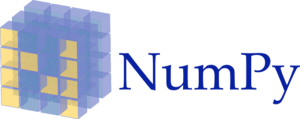NumPy or Numerical Python is one of the most commonly used Python libraries. The main feature of this library is its support for multi-dimensional arrays for mathematical and logical operations. Functions provided by NumPy can be used for indexing, sorting, reshaping and conveying images and sound waves as an array of real numbers in multi-dimension.

Here’s a list of features of NumPy:

1. Perform simple to complex mathematical and scientific computations
2. Strong support for multi-dimensional array objects and a collection of functions and methods to process the array elements
3. Fourier transformations and routines for data manipulation
4. Perform linear algebra computations, which are necessary for Machine Learning algorithms such as Linear Regression, Logistic Regression, Naive Bayes and so on.

## SciPy

Built on top of NumPy, the SciPy library is a collective of sub-packages which help in solving the most basic problems related to statistical analysis. SciPy library is used to process the array elements defined using the NumPy library, so it is often used to compute mathematical equations that cannot be done using NumPy.Here’s a list of features of SciPy:

• It works alongside NumPy arrays to provide a platform that provides numerous mathematical methods like, numerical integration and optimization.
• It has a collection of sub-packages that can be used for vector quantization, Fourier transformation, integration, interpolation and so on.
• Provides a fully-fledged stack of Linear Algebra functions which are used for more advanced computations such as clustering using the k-means algorithm and so on.
• Provides support for signal processing, data structures, and numerical algorithms, creating sparse matrices, and so on.

## Pandas

Pandas is another important statistical library mainly used in a wide range of fields including, statistics, finance, economics, data analysis and so on. The library relies on the NumPy array for the purpose of processing pandas data objects. NumPy, Pandas, and SciPy are heavily dependent on each other for performing scientific computations, data manipulation and so on.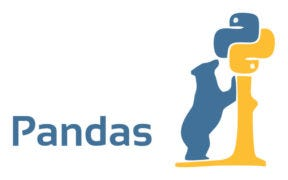I’m often asked to choose the best among Pandas, NumPy and SciPy, however, I prefer using all of them because they are heavily dependent on each other. Pandas is one of the best libraries for processing huge chunks of data, whereas NumPy has excellent support for multi-dimensional arrays and Scipy, on the other hand, provides a set of sub-packages that perform a majority of the statistical analysis tasks.

Here’s a list of features of Pandas:

• Creates fast and effective DataFrame objects with pre-defined and customized indexing.
• It can be used to manipulate large data sets and perform subsetting, data slicing, indexing and so on.
• Provides inbuilt features for creating Excel charts and performing complex data analysis tasks, such as descriptive statistical analysis, data wrangling, transformation, manipulation, visualization and so on.
• Provides support for manipulating Time Series data

## StatsModels

Built on top of NumPy and SciPy, the StatsModels Python package is the best for creating statistical models, data handling and model evaluation. Along with using NumPy arrays and scientific models from the SciPy library, it also integrates with Pandas for effective data handling. This library is famously known for statistical computations, statistical testing, and data exploration.

Here’s a list of features of StatsModels:

• Best library to perform statistical tests and hypothesis testing which are not found in NumPy and SciPy libraries.
• Provides the implementation of R-style formulas for better statistical analysis. It is more affiliated to the R language which is often used by statisticians.
• It is often used to implement Generalised Linear Models (GLM) and Ordinary least-square Linear Regression (OLM) models due it’s vast support for statistical computations.
• Statistical testing including hypothesis testing (Null Theory) is done using the StatsModels library.

So these were the most commonly used and the most effective Python libraries for statistical analysis. Now let’s get to the data visualization part in Data Science and Machine Learning.

# Python Libraries For Data Visualization

A picture speaks more than a thousand words. We’ve all heard of this quote in terms of art, however, it also holds true for Data Science and Machine Learning. Reputed Data Scientists and Machine Learning Engineers know the power of data visualization, that’s why Python provides tons of libraries for the sole purpose of visualization.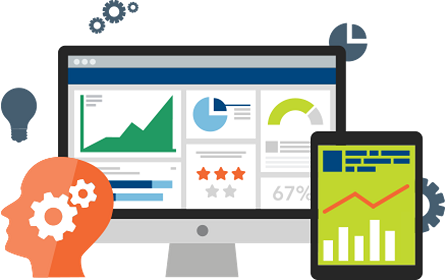Data Visualization is all about expressing the key insights from data, effectively through graphical representations. It includes the implementation of graphs, charts, mind maps, heat-maps, histograms, density plots, etc, to study the correlations between various data variables.

In this article, we’ll be focusing on the best Python data visualization packages that provide in-built functions to study the dependencies between various data features.

Here’s a list of the top Python libraries for data visualization:
Matplotlib

1. Seaborn
2. Plotly
3. Bokeh

## Matplotlib

Matplotlib is the most basic data visualization package in Python. It provides support for a wide variety of graphs such as histograms, bar charts, power spectra, error charts, and so on. It is a 2 Dimensional graphical library which produces clear and concise graphs that are essential for Exploratory Data Analysis (EDA).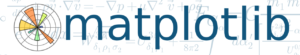Here’s a list of features of Matplotlib:

• Matplotlib makes it extremely easy to plot graphs by providing functions to choose appropriate line styles, font styles, formatting axes and so on.
• The graphs created help you get a clear understanding of the trends, patterns, and to make correlations. They’re typically instruments for reasoning about quantitative information.
• It contains the Pyplot module that provides an interface very similar to the MATLAB user interface. This is one of the best features of the matplotlib package.
• Provides an object-oriented API module for integrating graphs into applications using GUI tools like Tkinter, wxPython, Qt, etc.

## Seaborn

The Matplotlib library forms the base of the Seaborn library. In comparison to Matplotlib, Seaborn can be used to create more appealing and descriptive statistical graphs. Along with extensive supports for data visualization, Seaborn also comes with an inbuilt data set oriented API for studying the relationships between multiple variables.Here’s a list of features of Seaborn:

• Provides options for analyzing and visualizing univariate and bivariate data points and for comparing the data with other subsets of data.
• Support for automated statistical estimation and graphical representation of linear regression models for various kinds of target variables.
• Builds complex visualizations for structuring multi-plot grids by providing functions that perform high-level abstractions.
• Comes with numerous built-in themes for styling and creating matplotlib graphs

## Plotly

Ploty is one of the most well know graphical Python libraries. It provides interactive graphs for understanding the dependencies between target and predictor variables. It can be used to analyze and visualize statistical, financial, commerce and scientific data to produce clear and concise graphs, sub-plots, heatmaps, 3D charts and so on.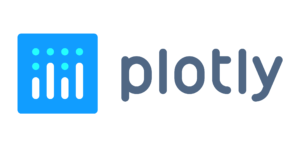Here’s a list of features that makes Ploty one of the best visualization libraries:

• It comes with more than 30 chart types, inclusive of 3D charts, scientific and statistical graphs, SVG maps, and so on for a well-defined visualization.
• With Ploty’s Python API, you can create public/ private dashboards that consist of plots, graphs, text and web images.
• Visualizations created using Ploty are serialized in the JSON format, due to which you can easily access them on different platforms like R, MATLAB, Julia, etc.
• It comes with an in-built API called Plotly Grid that allows you to directly import data into the Ploty environment.

## Bokeh

One of the most interactive libraries in Python, Bokeh can be used to build descriptive graphical representations for web browsers. It can easily process humungous datasets and build versatile graphs that help in performing extensive EDA. Bokeh provides the most well-defined functionality to build interactive plots, dashboards, and data applications.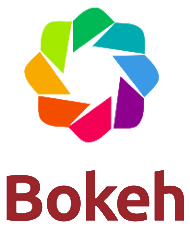Here’s a list of features of Bokeh:

• Helps you create complex statistical graphs quickly with the use of simple commands
• Supports outputs in the form of HTML, notebook, and server. It also supports multiple language bindings including, R, Python, Lua, Julia, etc.
• Flask and Django are also integrated with Bokeh, hence you can express visualizations on these apps as well
• It provides support to transform visualization written in other libraries like matplotlib, seaborn, ggplot, etc

So these were the most useful Python libraries for data visualization. Now let’s discuss the top Python libraries for implementing the whole Machine Learning process.

# Python Libraries For Machine Learning

Creating Machine Learning models that can accurately predict the outcome or solve a certain problem is the most important part of any Data Science project.

Implementing Machine Learning, Deep Learning, etc, involves coding 1000s of lines of code and this can become more cumbersome when you want to create models that solve complex problems through Neural Networks. But thankfully we don’t have to code any algorithms because Python comes with several packages just for the purpose of implementing Machine Learning techniques and algorithms.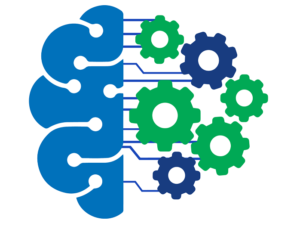In this blog, we’ll be focusing on the top Machine Learning packages that provide in-built functions to implement all the Machine Learning algorithms.

Here’s a list of the top Python libraries for Machine Learning:

1. Scikit-learn
2. XGBoost
3. Eli5

## Scikit-learn

One of the most useful Python libraries, Scikit-learn is the best library for data modeling and model evaluation. It comes with tons and tons of functions for the sole purpose of creating a model. It contains all the Supervised and Unsupervised Machine Learning algorithms and it also comes with well-defined functions for Ensemble Learning and Boosting Machine Learning.Here’s a list of features of Scikit-learn:

• Provides a set of standard datasets to help you get started with Machine Learning. For example, the famous Iris dataset and the Boston House Prices dataset are a part of the Scikit-learn library.
• In-built methods to carry out both Supervised and Unsupervised Machine Learning. This includes solving, clustering, classification, regression, and anomaly detection problems.
• Comes with in-built functions for feature extraction and feature selection which help in identifying the significant attributes in the data.
• It provides methods to perform cross-validation for estimating the performance of the model and also comes with functions for parameter tuning in order to improve the model performance.

## XGBoost

XGBoost which stands for Extreme Gradient Boosting is one of the best Python packages for performing Boosting Machine Learning. Libraries such as LightGBM and CatBoost are also equally equipped with well-defined functions and methods. This library is built mainly for the purpose of implementing gradient boosting machines which are used to improve the performance and accuracy of Machine Learning Models.Here are some of its key features:

• The library was originally written in C++, it is considered to be one of the fastest and effective libraries to improve the performance of Machine Learning models.
• The core XGBoost algorithm is parallelizable and it can effectively use the power of multi-core computers. This also makes the library strong enough to process massive data sets and work across a network of data sets.
• Provides internal parameters for performing cross-validation, parameter tuning, regularization, handling missing values, and also provides scikit-learn compatible APIs.
• This library is often used in the top Data Science and Machine Learning competitions since it has consistently proven to outperform other algorithms.

## ElI5

ELI5 is another Python library that is mainly focused on improving the performance of Machine Learning models. This library is relatively new and is usually used alongside the XGBoost, LightGBM, CatBoost and so on to boost the accuracy of Machine Learning models.

Here are some of its key features:

• Provides integration with Scikit-learn package to express feature importances and explain predictions of decision trees and tree-based ensembles.
• It analyzes and explains the predictions made by XGBClassifier, XGBRegressor, LGBMClassifier, LGBMRegressor, CatBoostClassifier, CatBoostRegressor and catboost.CatBoost.
• It provides support for implementing several algorithms in order to inspect black-box models which include the TextExplainer module that allows you to explain predictions made by text classifiers.
• It helps in analyzing weights and predictions of the scikit-learn General Linear Models (GLM) which include the linear regressors and classifiers.

# Python Libraries For Deep Learning

The biggest advancements in Machine Learning and Artificial Intelligence is been through Deep Learning. With the introduction to Deep Learning, it is now possible to build complex models and process humungous data sets. Thankfully, Python provides the best Deep Learning packages that help in building effective Neural Networks.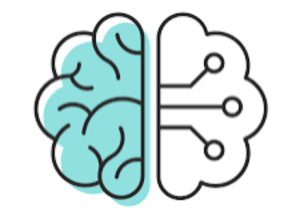In this blog, we’ll be focusing on the top Deep Learning packages that provide in-built functions to implement convoluted Neural Networks.

Here’s a list of the top Python libraries for Deep Learning:

1. TensorFlow
2. Pytorch
3. Keras

## Tensorflow

One of the best Python libraries for Deep Learning, TensorFlow is an open-source library for dataflow programming across a range of tasks. It is a symbolic math library that is used for building strong and precise neural networks. It provides an intuitive multiplatform programming interface which is highly-scalable over a vast domain of fields.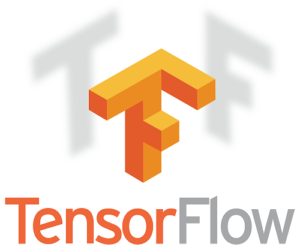Here are some key features of TensorFlow:

• It allows you to build and train multiple neural networks which help to accommodate large-scale projects and data sets.
• Along with support for Neural Networks, it also provides functions and methods to perform statistical analysis. For example, it comes with in-built functions for creating probabilistic models and Bayesian Networks such as Bernoulli, Chi2, Uniform, Gamma, etc.
• The library provides layered components that perform layered operations on weights and biases and also improve the performance of the model by implementing regularization techniques such as batch normalization, dropout, etc.
• It comes with a Visualizer called TensorBoard that creates interactive graphs and visuals to understand the dependencies of data features.

## Pytorch

Pytorch is an open-source, Python-based scientific computing package that is used to implement Deep Learning techniques and Neural Networks on large datasets. This library is actively used by Facebook to develop neural networks that help in various tasks such as face recognition and auto-tagging.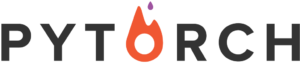Here are some key features of Pytorch:

• Provides easy to use APIs to integrate with other data science and Machine Learning frameworks.
• Like NumPy, Pytorch provides multi-dimensional arrays called Tensors, that unlike NumPy, can even be used on a GPU.
• Not only can it be used to model large-scale neural networks it also provides an interface, with more than 200+ mathematical operations for statistical analysis.
• Create Dynamic Computation Graphs that build-up dynamic graphs at every point of code execution. These graphs help in time series analysis while forecasting sales in real-time.

## Keras

Keras is considered as one of the best Deep Learning libraries in Python. It provides full support for building, analyzing, evaluating and improving Neural Networks. Keras is built on top of Theano and TensorFlow Python libraries which provides additional features to build complex and large-scale Deep Learning models.Here are some key features of Keras:

• Provides support to build all types of Neural Networks, i.e., fully connected, convolutional, pooling, recurrent, embedding, etc. For large data sets and problems, these models can further be combined to create a full-fledged Neural Network
• It has in-built functions to perform neural network computations such as defining layers, objectives, activation functions, optimizers and a host of tools to make working with image and text data easier.
• It comes with several pre-processed datasets and trained models including, MNIST, VGG, Inception, SqueezeNet, ResNet, etc.
• It is easily extensible and provides support to add new modules which include functions and methods.

# Python Libraries For Natural Language Processing

Have you ever wondered how Google so aptly predicts what you’re searching for? The technology behind Alexa, Siri, and other Chatbots is Natural Language Processing. NLP has played a huge role in designing AI-based systems that help in describing the interaction between human language and computers.In this blog, we’ll be focusing on the top Natural Language Processing packages that provide in-built functions to implement high-level AI-based systems.

Here’s a list of the top Python libraries for Natural Language Processing:

1. NLTK
2. SpaCy
3. Gensim

## NLTK (Natural Language ToolKit)

NLTK is considered to be the best Python package for analyzing human language and behavior. Preferred by most of the Data Scientists, the NLTK library provides easy-to-use interfaces containing over 50 corpora and lexical resources that help in describing human interactions and building AI-Based systems such as recommendation engines.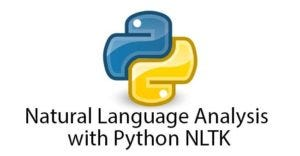Here are some key features of the NLTK library:

• Provides a suite of data and text processing methods for classification, tokenization, stemming, tagging, parsing, and semantic reasoning for text analysis.
• Contains wrappers for industrial-level NLP libraries to build convoluted systems that help in text classification and finding behavioral trends and patterns in human speech
• It comes with a comprehensive guide that describes the implementation of computational linguistics and a complete API documentation guide that helps all the newbies to get started with NLP.
• It has a huge community of users and professionals that provide comprehensive tutorials and quick guides to learn how computational linguistics can be carried out using Python.

## spaCy

spaCy is a free, open-source Python library for implementing advanced Natural Language Processing (NLP) techniques. When you’re working with a lot of text it is important that you understand the morphological meaning of the text and how it can be classified to understand human language. These tasks can be easily achieved through spaCY.Here are some key features of the spaCY library:

• Along with linguistic computations, spaCy provides separate modules to build, train and test statistical models that will better help you understand the meaning of a word.
• Comes with a variety of built-in linguistic annotations to help you analyze the grammatical structure of a sentence. This not only helps in understanding the test, but it also assists in finding the relations between different words in a sentence.
• It can be used to apply tokenization on complex, nested tokens that contain abbreviations and multiple punctuation marks.
• Along with being extremely robust and fast, spaCy provides support for 51+ languages.

## Gensim

Gensim is another open-source Python package modeled to extract semantic topics from large documents and texts to process, analyze and predict human behavior through statistical models and linguistic computations. It has the capability to process humungous data, irrespective of whether the data is raw and unstructured.Here are some key features of Genism:

• It can be used to build models that can effectively classify documents by understanding the statistical semantic of each word.
• It comes with text processing algorithms such as Word2Vec, FastText, Latent Semantic Analysis, etc that study the statistical co-occurrence patterns in the document to filter out unnecessary words and build a model with just the significant features.
• Provides I/O wrappers and readers that can import and support a vast range of data formats.
• It comes with simple and intuitive interfaces that can easily be used by beginners. The API learning curve is also quite low which explains why a lot of developers like this library.

If you wish to check out more articles on the market’s most trending technologies like Artificial Intelligence, DevOps, Ethical Hacking, then you can refer to Edureka’s official site.

Do look out for other articles in this series which will explain the various other aspects of Deep Learning.